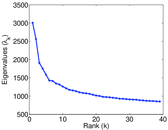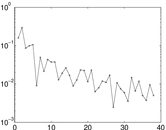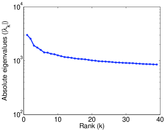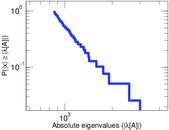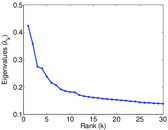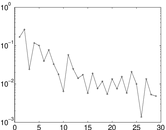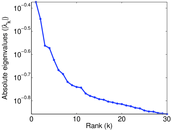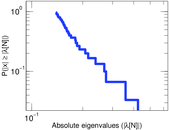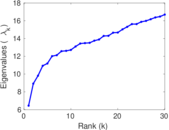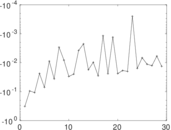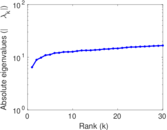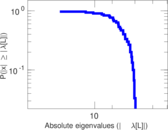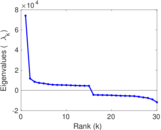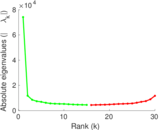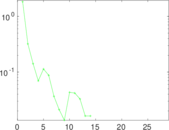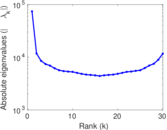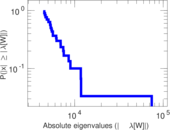# Jester 100

This weighted network contains information about how users rated a total ammount of 100 jokes. Not every user rated every joke. Rating values are continuous values between −10 and +10. An edge shows that a user has rated a joke. Left nodes are users and right nodes are jokes.

 Code `J1` Internal name `jester1` Name Jester 100 Data source http://eigentaste.berkeley.edu/dataset/ AvailabilityDataset is available for download Consistency checkDataset passed all tests Category Rating network Dataset timestamp 1999 ⋯ 2003 Node meaning User, joke Edge meaning Rating Network formatBipartite, undirected Edge type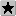Ratings, no multiple edges

## Statistics

 Size n = 73,521 Left size n1 = 73,421 Right size n2 = 100 Volume m = 4,136,360 Wedge count s = 102,339,759,934 Claw count z = 1,907,263,353,344,646 Cross count x = 2.893 18 × 1019 Square count q = 2,599,984,203,310 4-Tour count T4 = 21,209,254,824,380 Maximum degree dmax = 73,413 Maximum left degree d1max = 100 Maximum right degree d2max = 73,413 Average degree d = 112.522 Average left degree d1 = 56.337 6 Average right degree d2 = 41,363.6 Fill p = 0.563 376 Size of LCC N = 73,521 Diameter δ = 4 50-Percentile effective diameter δ0.5 = 1.499 62 90-Percentile effective diameter δ0.9 = 1.900 42 Median distance δM = 2 Mean distance δm = 1.999 22 Gini coefficient G = 0.645 907 Balanced inequality ratio P = 0.269 422 Left balanced inequality ratio P1 = 0.385 244 Right balanced inequality ratio P2 = 0.404 071 Relative edge distribution entropy Her = 0.756 826 Power law exponent γ = 1.849 21 Tail power law exponent γt = 2.091 00 Degree assortativity ρ = −0.413 704 Degree assortativity p-value pρ = 0.000 00 Spectral norm α = 3,007.63 Algebraic connectivity a = 8.924 26 Spectral separation |λ1[A] / λ2[A]| = 1.172 23 Negativity ζ = 0.455 631 Algebraic conflict ξ = 14.999 6 Spectral signed frustration φ = 0.033 325 9

## Plots

### Fruchterman–Reingold graph drawing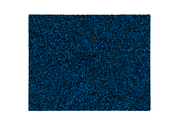### Degree distribution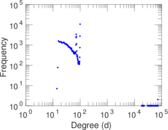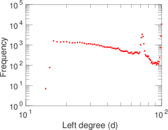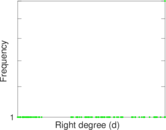### Cumulative degree distribution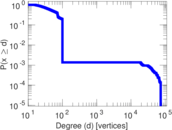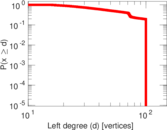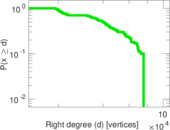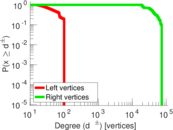### Lorenz curve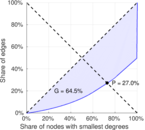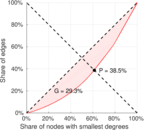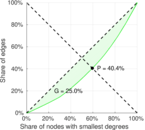### Spectral distribution of the adjacency matrix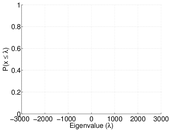### Spectral distribution of the normalized adjacency matrix### Spectral distribution of the Laplacian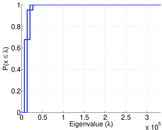### Spectral graph drawing based on the adjacency matrix### Spectral graph drawing based on the Laplacian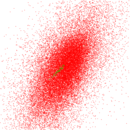### Spectral graph drawing based on the normalized adjacency matrix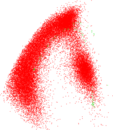### Degree assortativity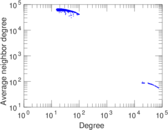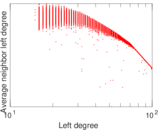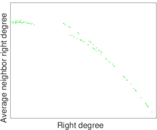### Zipf plot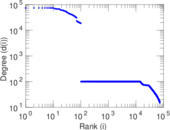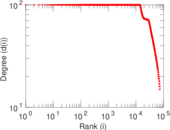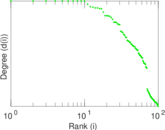### Hop distribution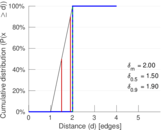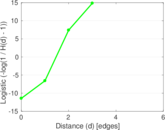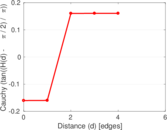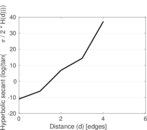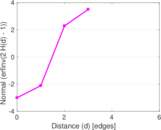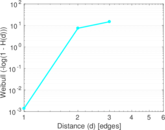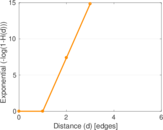### Double Laplacian graph drawing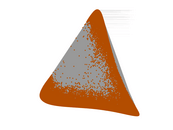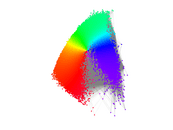### Delaunay graph drawing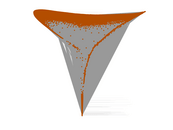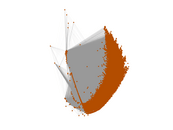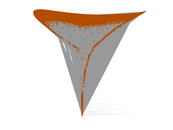### Item rating evolution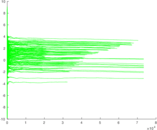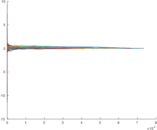### Edge weight/multiplicity distribution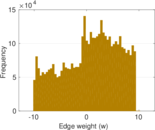### Matrix decompositions plots